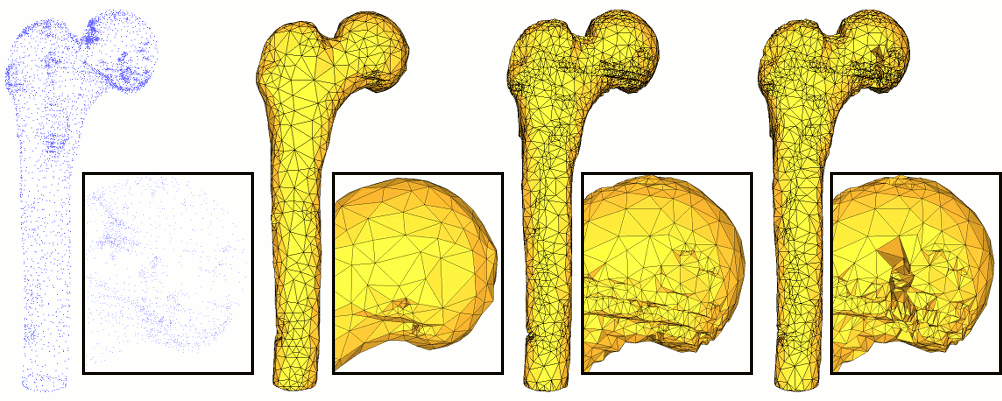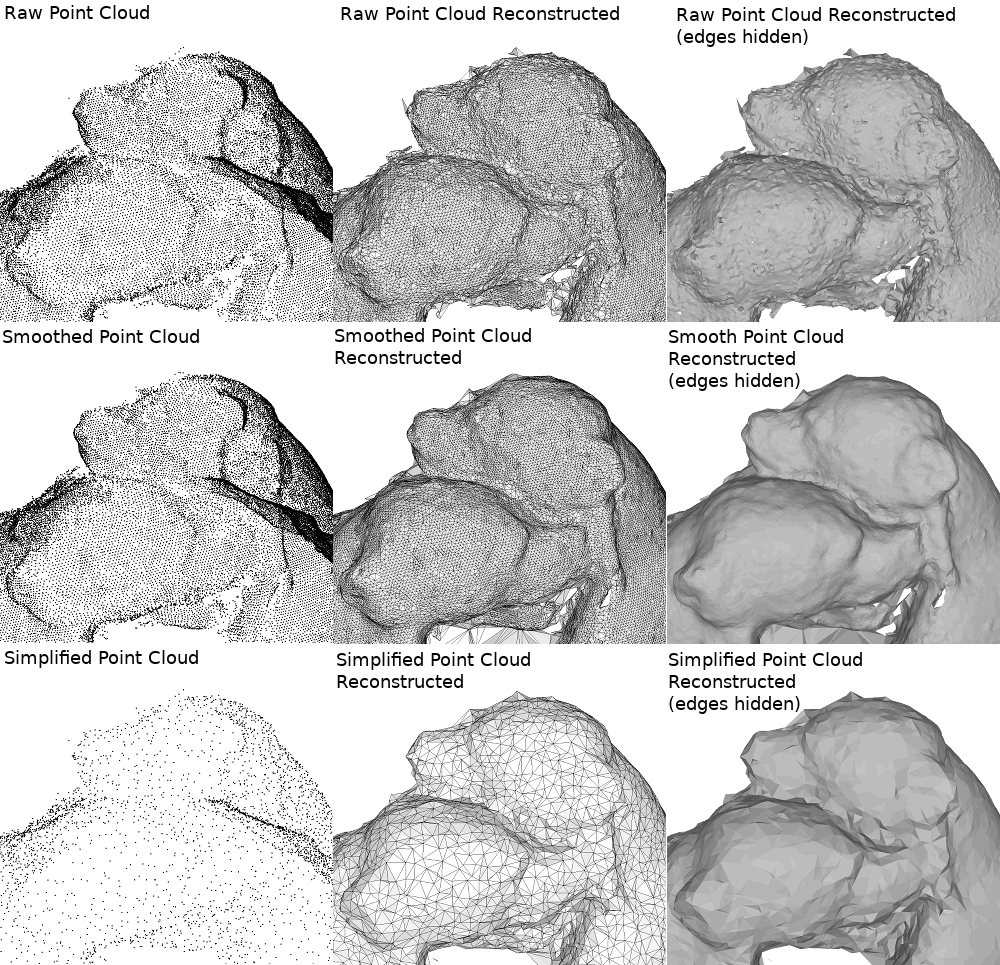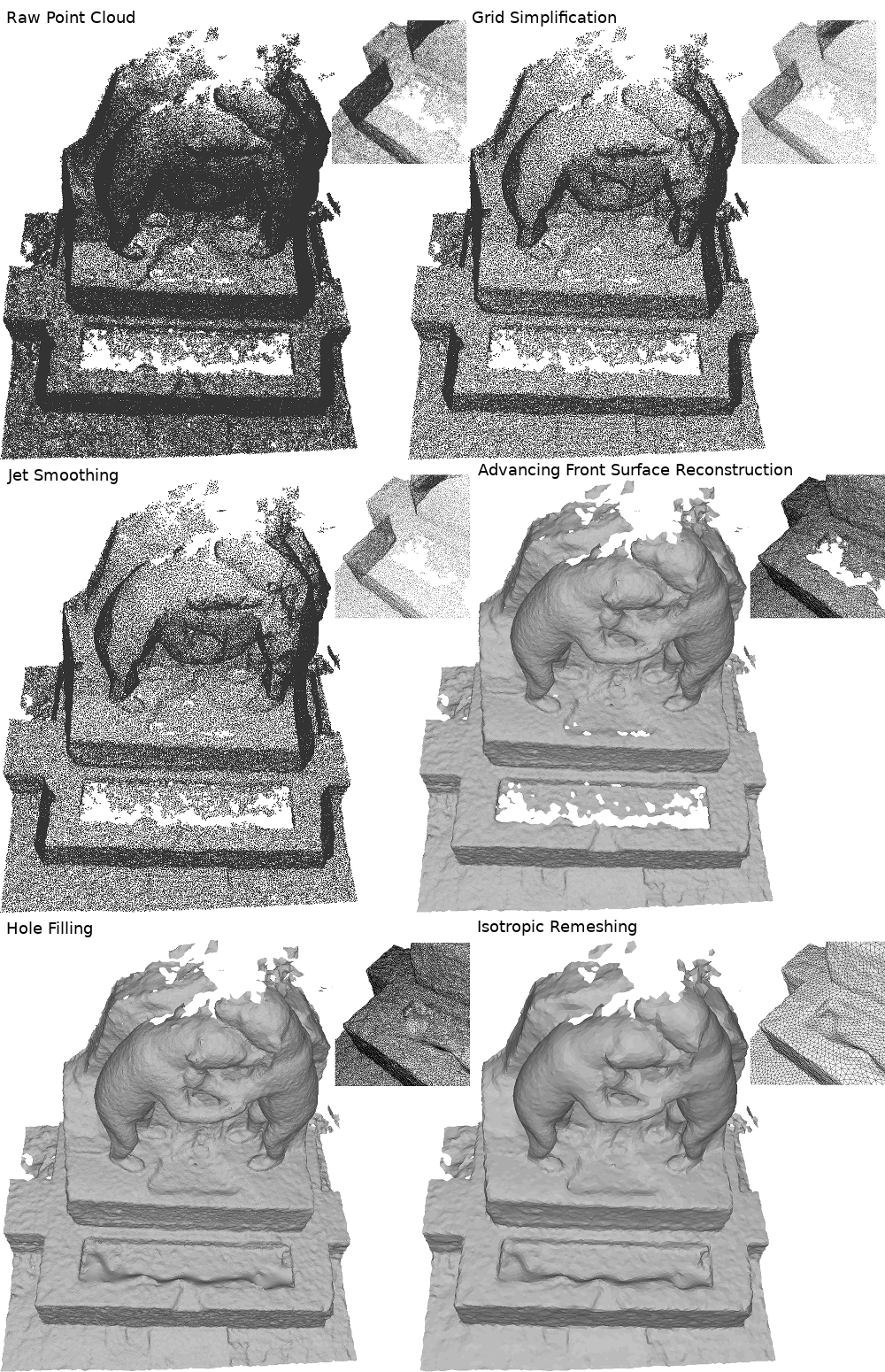CGAL 5.3 - Manual
Surface Reconstruction from Point Clouds

Surface reconstruction from point clouds is a core topic in geometry processing . It is an ill-posed problem: there is an infinite number of surfaces that approximate a single point cloud and a point cloud does not define a surface in itself. Thus additional assumptions and constraints must be defined by the user and reconstruction can be achieved in many different ways. This tutorial provides guidance on how to use the different algorithms of CGAL to effectively perform surface reconstruction.

# Which algorithm should I use?

CGAL offers three different algorithms for surface reconstruction:

Because reconstruction is an ill-posed problem, it must be regularized via prior knowledge. Differences in prior lead to different algorithms, and choosing one or the other of these methods is dependent on these priors. For example, Poisson always generates closed shapes (bounding a volume) and requires normals but does not interpolate input points (the output surface does not pass exactly through the input points). The following table lists different properties of the input and output to help the user choose the method best suited to each problem:

Are normals required? Yes No No
Is noise handled? Yes By preprocessing Yes
Is variable sampling handled? Yes Yes By preprocessing
Are input points exactly on the surface? No Yes Yes
Is the output always closed? Yes No No
Is the output always smooth? Yes No No
Is the output always manifold? Yes Yes Optional
Is the output always orientable? Yes Yes OptionalFigure 0.1 Comparison of reconstruction methods applied to the same input (full shape and close-up). From left to right: original point cloud; Poisson; advancing front; scale space.

More information on these different methods can be found on their respective manual pages and in Section Reconstruction.

# Pipeline Overview

This tutorial aims at providing a more comprehensive view of the possibilities offered by CGAL for dealing with point clouds, for surface reconstruction purposes. The following diagram shows an overview (not exhaustive) of common reconstruction steps using CGAL tools.

Figure 0.2 Pipeline Overview

We now review some of these steps in more detail.

The reconstruction algorithms in CGAL take a range of iterators on a container as input and use property maps to access the points (and the normals when they are required). Points are typically stored in plain text format (denoted as 'XYZ' format) where each point is separated by a newline character and each coordinate separated by a white space. Other formats available are 'OFF', 'PLY' and 'LAS'. CGAL provides functions to read such formats:

• read_XYZ()
• read_OFF()
• read_PLY()
• read_PLY_with_properties() to read additional PLY properties
• read_LAS()
• read_LAS_with_properties() to read additional LAS properties

CGAL also provides a dedicated container CGAL::Point_set_3 to handle point sets with additional properties such as normal vectors. In this case, property maps are easily handled as shown in the following sections. This structure also handles the stream operator to read point sets in any of the formats previously described. Using this method yields substantially shorter code, as can be seen on the following example:

Point_set points;
if (argc < 2)
{
std::cerr << "Usage: " << argv << " [input.xyz/off/ply/las]" << std::endl;
return EXIT_FAILURE;
}
const char* input_file = argv;
std::ifstream stream (input_file, std::ios_base::binary);
if (!stream)
{
std::cerr << "Error: cannot read file " << input_file << std::endl;
return EXIT_FAILURE;
}
stream >> points;
std::cout << "Read " << points.size () << " point(s)" << std::endl;
if (points.empty())
return EXIT_FAILURE;

# Preprocessing

Because reconstruction algorithms have some specific requirements that point clouds do not always meet, some preprocessing might be necessary to yield the best results.

Note that this preprocessing step is optional: when the input point cloud has no imperfections, reconstruction can be applied to it without any preprocessing.Figure 0.3 Comparison of advancing front reconstruction output using different preprocessing on the same input. The smooth point cloud was generated using jet smoothing; the simplified point cloud was generated using grid simplification.

## Outlier removal

Some acquisition techniques generate points which are far away from the surface. These points, commonly referred to as "outliers", have no relevance for reconstruction. Using the CGAL reconstruction algorithms on outlier-ridden point clouds produce overly distorted output, it is therefore strongly advised to filter these outliers before performing reconstruction.

CGAL::remove_outliers<CGAL::Sequential_tag>
(points,
24, // Number of neighbors considered for evaluation
points.parameters().threshold_percent (5.0)); // Percentage of points to remove
std::cout << points.number_of_removed_points()
<< " point(s) are outliers." << std::endl;
// Applying point set processing algorithm to a CGAL::Point_set_3
// object does not erase the points from memory but place them in
// the garbage of the object: memory can be freeed by the user.
points.collect_garbage();

## Simplification

Some laser scanners generate points with widely variable sampling. Typically, lines of scan are very densely sampled but the gap between two lines of scan is much larger, leading to an overly massive point cloud with large variations of sampling density. This type of input point cloud might generate imperfect output using algorithms which, in general, only handle small variations of sampling density.

CGAL provides several simplification algorithms. In addition to reducing the size of the input point cloud and therefore decreasing computation time, some of them can help making the input more uniform. This is the case of the function grid_simplify_point_set() which defines a grid of a user-specified size and keeps one point per occupied cell.

// Compute average spacing using neighborhood of 6 points
double spacing = CGAL::compute_average_spacing<CGAL::Sequential_tag> (points, 6);
// Simplify using a grid of size 2 * average spacing
CGAL::grid_simplify_point_set (points, 2. * spacing);
std::cout << points.number_of_removed_points()
<< " point(s) removed after simplification." << std::endl;
points.collect_garbage();

## Smoothing

Although reconstructions via 'Poisson' or 'Scale space' handle noise internally, one may want to get tighter control over the smoothing step. For example, a slightly noisy point cloud can benefit from some reliable smoothing algorithms and be reconstructed via 'Advancing front' which provides relevant properties (oriented mesh with boundaries).

Two functions are provided to smooth a noisy point cloud with a good approximation (i.e. without degrading curvature, for example):

• jet_smooth_point_set()
• bilateral_smooth_point_set()

These functions directly modify the container:

CGAL::jet_smooth_point_set<CGAL::Sequential_tag> (points, 24);

## Normal Estimation and Orientation

Poisson Surface Reconstruction requires points with oriented normal vectors. To apply the algorithm to a raw point cloud, normals must be estimated first, for example with one of these two functions:

• pca_estimate_normals()
• jet_estimate_normals()

PCA is faster but jet is more accurate in the presence of high curvatures. These function only estimates the direction of the normals, not their orientation (the orientation of the vectors might not be locally consistent). To properly orient the normals, the following functions can be used:

• mst_orient_normals()
• scanline_orient_normals()

The first one uses a minimum spanning tree to consistently propagate the orientation of normals in an increasingly large neighborhood. In the case of data with many sharp features and occlusions (which are common in airborne LIDAR data, for example), the second algorithm may produce better results: it takes advantage of point clouds which are ordered into scanlines to estimate the line of sight of each point and thus to orient normals accordingly.

Notice that these can also be used directly on input normals if their orientation is not consistent.

CGAL::jet_estimate_normals<CGAL::Sequential_tag>
(points, 24); // Use 24 neighbors
// Orientation of normals, returns iterator to first unoriented point
typename Point_set::iterator unoriented_points_begin =
CGAL::mst_orient_normals(points, 24); // Use 24 neighbors
points.remove (unoriented_points_begin, points.end());

# Reconstruction

## Poisson

Poisson reconstruction consists in computing an implicit function whose gradient matches the input normal vector field: this indicator function has opposite signs inside and outside of the inferred shape (hence the need for closed shapes). This method thus requires normals and produces smooth closed surfaces. It is not appropriate if the surface is expected to interpolate the input points. On the contrary, it performs well if the aim is to approximate a noisy point cloud with a smooth surface.

(points.begin(), points.end(),
points.point_map(), points.normal_map(),
output_mesh, spacing);

Advancing front is a Delaunay-based approach which interpolates a subset of the input points. It generates triples of point indices which describe the triangular facets of the reconstruction: it uses a priority queue to sequentially pick the Delaunay facet the most likely to be part of the surface, based on a size criterion (to favor the small facets) and an angle criterion (to favor smoothness). Its main virtue is to generate oriented manifold surfaces with boundaries: contrary to Poisson, it does not require normals and is not bound to reconstruct closed shapes. However, it requires preprocessing if the point cloud is noisy.

The Advancing Front package provides several ways of constructing the function. Here is a simple example:

typedef std::array<std::size_t, 3> Facet; // Triple of indices
std::vector<Facet> facets;
// The function is called using directly the points raw iterators
points.points().end(),
std::back_inserter(facets));
std::cout << facets.size ()
<< " facet(s) generated by reconstruction." << std::endl;

## Scale Space

Scale space reconstruction aims at producing a surface which interpolates the input points (interpolant) while offering some robustness to noise. More specifically, it first applies several times a smoothing filter (such as Jet Smoothing) to the input point set to produce a scale space; then, the smoothest scale is meshed (using for example the Advancing Front mesher); finally, the resulting connectivity between smoothed points is propagated to the original raw input point set. This method is the right choice if the input point cloud is noisy but the user still wants the surface to pass exactly through the points.

(points.points().begin(), points.points().end());
// Smooth using 4 iterations of Jet Smoothing
// Mesh with the Advancing Front mesher with a maximum facet length of 0.5

# Output and Postprocessing

Each of these methods produce a triangle mesh stored in different ways. If this output mesh is hampered by defects such as holes or self-intersections, CGAL provide several algorithms to post-process it (hole filling, remeshing, etc.) in the package Polygon Mesh Processing.

We do not discuss these functions here as there are many postprocessing possibilities whose relevance strongly depends on the user's expectations on the output mesh.

The mesh (postprocessed or not) can easily be saved in the PLY format (here, using the binary variant):

std::ofstream f ("out.ply", std::ios_base::binary);
CGAL::IO::write_PLY(f, output_mesh);
f.close ();

A polygon soup can also be saved in the OFF format by iterating on the points and faces:

std::ofstream f ("out.off");
f << "OFF" << std::endl << points.size () << " "
<< reconstruct.number_of_facets() << " 0" << std::endl;
for (Point_set::Index idx : points)
f << points.point (idx) << std::endl;
for (const auto& facet : CGAL::make_range (reconstruct.facets_begin(), reconstruct.facets_end()))
f << "3 "<< facet << std::endl;
f.close ();

Finally, if the polygon soup can be converted into a polygon mesh, it can also be saved directly in the OFF format using the stream operator:

// copy points for random access
std::vector<Point_3> vertices;
vertices.reserve (points.size());
std::copy (points.points().begin(), points.points().end(), std::back_inserter (vertices));
std::ofstream f ("out.off");
f << output_mesh;
f.close ();

# Full Code Example

All the code snippets used in this tutorial can be assembled to create a full algorithm pipeline (provided the correct includes are used). We give a full code example which achieves all the steps described in this tutorial. The reconstruction method can be selected by the user at runtime with the second argument.

#include <CGAL/Exact_predicates_inexact_constructions_kernel.h>
#include <CGAL/Point_set_3.h>
#include <CGAL/Point_set_3/IO.h>
#include <CGAL/remove_outliers.h>
#include <CGAL/grid_simplify_point_set.h>
#include <CGAL/jet_smooth_point_set.h>
#include <CGAL/jet_estimate_normals.h>
#include <CGAL/mst_orient_normals.h>
#include <CGAL/poisson_surface_reconstruction.h>
#include <CGAL/Scale_space_surface_reconstruction_3.h>
#include <CGAL/Scale_space_reconstruction_3/Jet_smoother.h>
#include <CGAL/Surface_mesh.h>
#include <CGAL/Polygon_mesh_processing/polygon_soup_to_polygon_mesh.h>
#include <cstdlib>
#include <vector>
#include <fstream>
// types
typedef Kernel::FT FT;
typedef Kernel::Point_3 Point_3;
typedef Kernel::Vector_3 Vector_3;
typedef Kernel::Sphere_3 Sphere_3;
int main(int argc, char*argv[])
{
Point_set points;
if (argc < 2)
{
std::cerr << "Usage: " << argv << " [input.xyz/off/ply/las]" << std::endl;
return EXIT_FAILURE;
}
const char* input_file = argv;
std::ifstream stream (input_file, std::ios_base::binary);
if (!stream)
{
std::cerr << "Error: cannot read file " << input_file << std::endl;
return EXIT_FAILURE;
}
stream >> points;
std::cout << "Read " << points.size () << " point(s)" << std::endl;
if (points.empty())
return EXIT_FAILURE;
CGAL::remove_outliers<CGAL::Sequential_tag>
(points,
24, // Number of neighbors considered for evaluation
points.parameters().threshold_percent (5.0)); // Percentage of points to remove
std::cout << points.number_of_removed_points()
<< " point(s) are outliers." << std::endl;
// Applying point set processing algorithm to a CGAL::Point_set_3
// object does not erase the points from memory but place them in
// the garbage of the object: memory can be freeed by the user.
points.collect_garbage();
// Compute average spacing using neighborhood of 6 points
double spacing = CGAL::compute_average_spacing<CGAL::Sequential_tag> (points, 6);
// Simplify using a grid of size 2 * average spacing
CGAL::grid_simplify_point_set (points, 2. * spacing);
std::cout << points.number_of_removed_points()
<< " point(s) removed after simplification." << std::endl;
points.collect_garbage();
CGAL::jet_smooth_point_set<CGAL::Sequential_tag> (points, 24);
unsigned int reconstruction_choice
= (argc < 3 ? 0 : atoi(argv));
if (reconstruction_choice == 0) // Poisson
{
CGAL::jet_estimate_normals<CGAL::Sequential_tag>
(points, 24); // Use 24 neighbors
// Orientation of normals, returns iterator to first unoriented point
typename Point_set::iterator unoriented_points_begin =
CGAL::mst_orient_normals(points, 24); // Use 24 neighbors
points.remove (unoriented_points_begin, points.end());
(points.begin(), points.end(),
points.point_map(), points.normal_map(),
output_mesh, spacing);
std::ofstream f ("out.ply", std::ios_base::binary);
CGAL::IO::write_PLY(f, output_mesh);
f.close ();
}
else if (reconstruction_choice == 1) // Advancing front
{
typedef std::array<std::size_t, 3> Facet; // Triple of indices
std::vector<Facet> facets;
// The function is called using directly the points raw iterators
points.points().end(),
std::back_inserter(facets));
std::cout << facets.size ()
<< " facet(s) generated by reconstruction." << std::endl;
// copy points for random access
std::vector<Point_3> vertices;
vertices.reserve (points.size());
std::copy (points.points().begin(), points.points().end(), std::back_inserter (vertices));
std::ofstream f ("out.off");
f << output_mesh;
f.close ();
}
else if (reconstruction_choice == 2) // Scale space
{
(points.points().begin(), points.points().end());
// Smooth using 4 iterations of Jet Smoothing
// Mesh with the Advancing Front mesher with a maximum facet length of 0.5
std::ofstream f ("out.off");
f << "OFF" << std::endl << points.size () << " "
<< reconstruct.number_of_facets() << " 0" << std::endl;
for (Point_set::Index idx : points)
f << points.point (idx) << std::endl;
for (const auto& facet : CGAL::make_range (reconstruct.facets_begin(), reconstruct.facets_end()))
f << "3 "<< facet << std::endl;
f.close ();
}
else // Handle error
{
std::cerr << "Error: invalid reconstruction id: " << reconstruction_choice << std::endl;
return EXIT_FAILURE;
}
return EXIT_SUCCESS;
}

# Full Pipeline Images

The following figure an example of a full reconstruction pipeline applied to a bear statue (courtesy EPFL Computer Graphics and Geometry Laboratory ). Two mesh processing algorithms (hole filling and isotropic remeshing) are also applied (refer to the chapter Polygon Mesh Processing for more information).Figure 0.4 Full reconstruction pipeline (with close-ups).